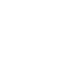# Question of the Day (SAT & ACT): Nov. 12, 2013

If you are reading this in an email you received from me, do not click the link to sat.collegeboard.org below. Use the link to my website that is farther down on the email.

If you are seeing this in my blog, do the SAT Question of the Day by clicking on this link:

http://sat.collegeboard.org/practice/sat-question-of-the-day?questionId=20131112&oq=1 (This link takes you to today’s question. If you use my archive, you will see the question related to my SAT explanation for that date.)

The answer is C.  Yes, you can do it the way that the test writer explains it.  You should read it because that explanation works for many students.  It is the traditional math teacher approach to the question.

Let’s see if there’s an easier way to do it.  I tried the answers and worked backwards because one of them has to be right (Pillar V).  There’s a couple of things about the structure of the SAT that makes this a more efficient way to do the problem for many students.  First, don’t forget the “cheat sheet” with the geometry equations at the beginning of the test section.  It has the equation you need.  For a cylinder, V=πr2h.  Second, the answers are organized in ascending or descending order; so, start with C.  (If C is wrong, you’ll know whether to go larger or smaller which means you’ve eliminated 3 answers for the price of 1!)   If the circumference is 6π, (check the cheat sheet for the equation to convert circumference to radius) then the radius is 3.  32 is 9 and 9 times the height, 6, is 54π.  All done.  For me, that was much faster than the “math teacher” method.

You need to see how many ways you can do the math problems.  Practice is going to help you decide which methods are the quickest for you to accurately get an answer.  For some students, the math teacher technique works just fine but for others it often slows them down.   Remember that there are no right ways to do the questions; there are only right answers!

Let’s see what the ACT folks have for us this morning.

Do you know how to interpolate and extrapolate?  You need to learn if you don’t already know.  The test writers like to see if you can calculate missing data points.  Just use the trend of the data to calculate a missing point within the range you are given, interpolate, or beyond the range, extrapolate. You should check out Video #10 if you want to learn more about the nature of the ACT Science Test.  You could also check out my free website.

The answer is B.  You can see that for every 5cm in depth you go, the pH goes down 0.5.  That makes the trend quite clear.  If you go to 35cm, 15cm deeper than the data you are given, then you have to go 15/5, 3, more steps in reducing the value of the pH.  3 times .5 is 1.5 and 5.0 (the last pH you are given) minus 1.5 is 3.5, the right answer.  Or you can just count.  35 would be 3 more steps on the depth chart since you are counting by 5.  3.5 would be three more steps in the pH column when you count down 0.5 3 more steps.

This is an example of what to do to best prepare for the Science Test.  Don’t bother reviewing science because they’ll give you all the science in the test booklet.  You need to practice reading and using data.  Get your hands on some old ACT tests and get to work practicing.

Have a great day.  Say something nice to somebody about how he or she is looking today.  It will cheer you both up!

The SAT & ACT Wizard